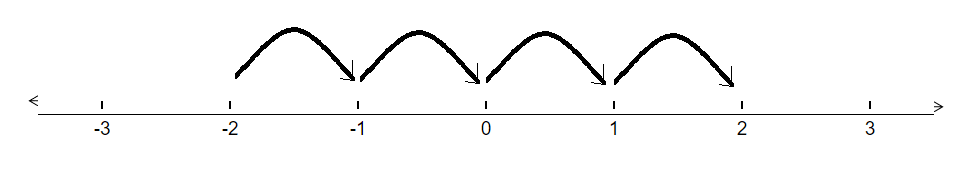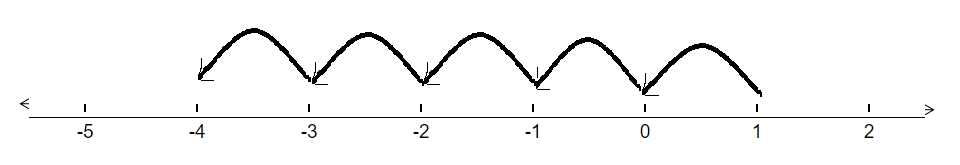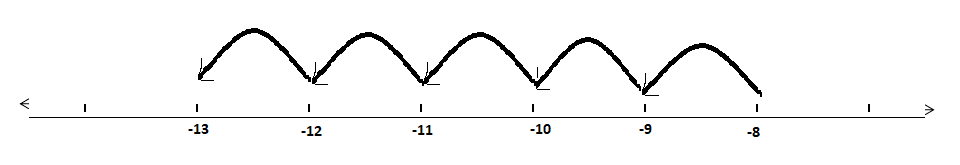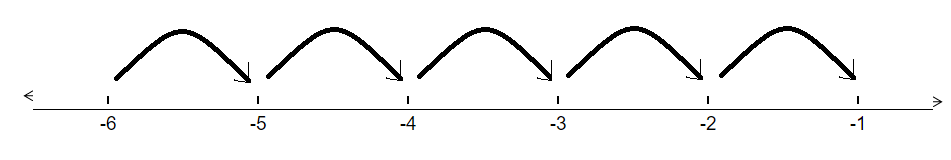# Draw a number line and answer the following:(a) Which number will we reach if we move 4 numbers to the right of $‚Äď 2$.(b) Which number will we reach if we move 5 numbers to the left of 1.(c) If we are at $‚Äď 8$ on the number line, in which direction should we move toreach $‚Äď 13$?(d) If we are at $‚Äď 6$ on the number line, in which direction should we move toreach $‚Äď 1$?

To do:

We have to draw a number line and answer the given questions.

Solution:

(a)Therefore,

If we move 4 numbers to the right of $-2$, we will reach 2.

(b)Therefore,

If we move 5 numbers to the left of 1, we will reach $-4$

(c)$-13$ lies to the left of $-8$ on the number line.

Therefore, we should move in the left direction

(d)$-1$ lies to the right of $-6$ on the number line.

Therefore, we should move in the right direction.